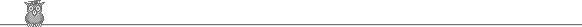### 27th USAMO 1998Problem A1

The sets {a1, a2, ... , a999} and {b1, b2, ... , b999} together contain all the integers from 1 to 1998. For each i, |ai - bi| = 1 or 6. For example, we might have a1 = 18, a2 = 1, b1 = 17, b2 = 7. Show that ∑1999 |ai - bi| = 9 mod 10.

Solution

If |ai - bi| = 6, then ai and bi have the same parity, so the set of such ai and bi contains an even number of odd numbers. But if |ai - bi| = 1, then ai and bi have opposite parity, so each such pair includes just one odd number. Hence if the number of such pairs is even, then the set of all such ai and bi also has an even number of odd numbers. But the total number of ai and bi which are odd is 999 which is odd. Hence the number of pairs with |ai - bi| = 1 must be odd, and hence the number of pairs with |ai - bi| = 6 must be even. Suppose it is 2k. Then ∑ |ai - bi| = (999 - 2k) 1 + 2k 6 = 999 + 10k = 9 mod 10.